### DatasheetsN° of component

## Rectification - How it Works (ART501E)

This article is part of our Electronic Course - Basic Electronics and Electronic Course - Power Electronics (*) where the reader can find additional information about the operation of diodes and rectifier circuits. In ART3971, we also have an explanation of how semiconductor diodes work.

(*) Soon in English

## Rectification

Higher current capacity and higher voltage diodes are basically used in rectification in power applications.

In our Basic Course, we explain how rectifier circuits work, however we will review the concepts, because we need to go further since in power applications it is common for them to be used in three phase circuits.

Reviewing the basic concepts, we know that in rectification the three ways to use diodes are:

## a) Half Wave Rectifier

In this circuit, in positive half cycles of the alternating voltage in the transformer secondary, the diode is polarized in forward, so as to present low resistance and let the current pass. However, in negative half-cycles, the diode is polarized in reverse and no current can pass, as shown in Figure 1.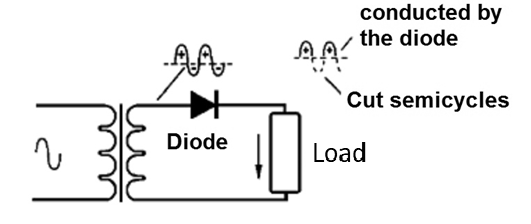Figure 1 - Conducting only positive half cycles

See then that the current only passes in the positive half cycles or on only one-way. This current, though circulating in one direction, is not a pure direct current.

It is formed by “pulses” which appear only at times when the diode is polarized in the direct direction. We say it is a "pulsating direct current".

If we reverse the diode, as the reader can see in Figure 2, we will have the current flow only in the negative half cycles and still a pulsating current, but reverse in the direction or polarity.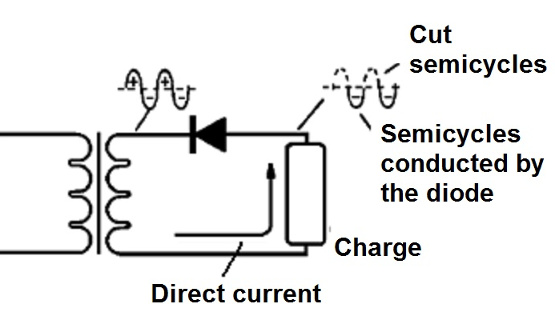Figure 2 - Letting the negative half cycles go through

Since only half of the alternating current, AC current, half cycles are driven in this process, we say it is a “half wave” rectification process.

## b) Full wave rectifier

One way to achieve greater efficiency in rectification or “direct current transformation” is by taking advantage of the two half-cycles.

This is possible if we use a transformer with a central socket and two diodes, connected as the reader can see in Figure 3.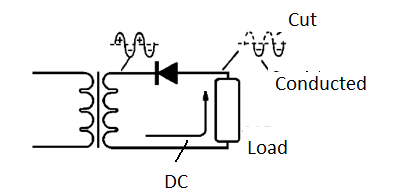Figure 3 - Using two diodes

When transformer terminal A is positive for CT, in positive half cycles, terminal B, at the same time, is negative for CT.

Thus, while in positive input half cycles, diode D1 is polarized in the direct way, diode D2 will be polarized in the reverse way. It then leads diode D1, as we can see in Figure 4.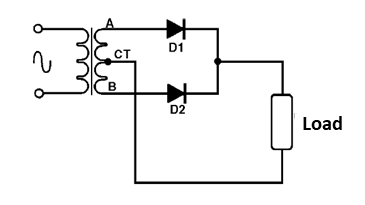Figure 4 - Driving on the positive half cycles

In the negative half icycle of the input voltage, things are reversed. While A is negative related to CT, B is positive, so that D1 will be inversely polarized and D2 directly. It drives D2 , as shown in Figure 5, and the load receives its power.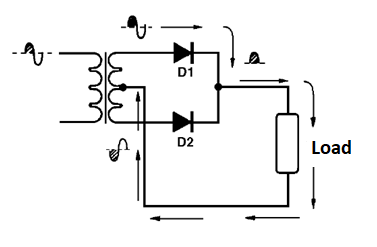Figure 5 - Driving negative half cycles

In other words, in this process, the full wave rectification is harnessed and is therefore called “full wave rectification”.

Note that the transformer allows the negative half cycle to be “inverted” to be taken advantage of as well.

Of course, this ratification process presents itself twice as efficient as the previous one, and therefore has many advantages for use.

Note that we still have a pulsating direct current, DC current, in the load, although it has “minor” variations than in the previous case.

## c) Bridge rectifier

One way to achieve full wave rectification using a common transformer, i.e., single secondary, without a center socket, is possible using 4 diodes, i.e., a diode bridge as shown in the Figure 6.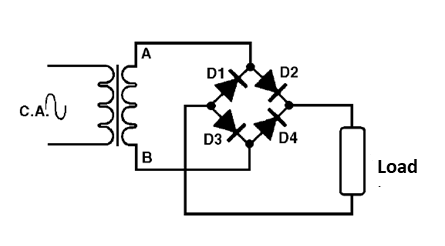Figure 6 - Using a diode bridge (Graetz Bridge)

Let's see how this system called “bridge rectification” works: In positive half cycles, terminal A of the transformer is positive in relation to terminal B.

This way, the diodes D2 and D3 are polarized in the direct direction, driving the current, it can be seen in Figure 7.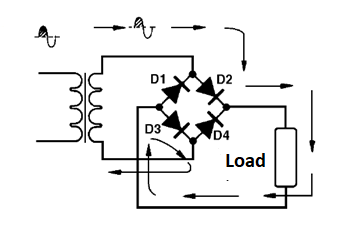Figure 7 - Bridge driving on positive half cycles

In negative half cycles, diodes D1 and D4 are polarized in the direct direction, which then drive the current as shown in Figure 8.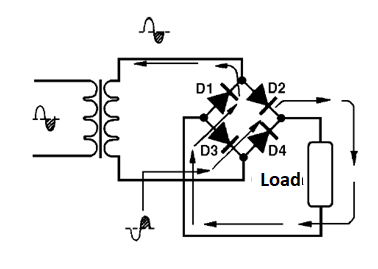Figure 8 - Current in Negative Half cycles

It is important to note that in this system, the current in each half cycle passes through two diodes instead of one, as in the others. This means that we have a larger voltage drop in the rectifying system.

So while in the full-wave system we 'only lose' 0.6 V in the silicon diode, in this system we 'lose' 1.2 V. Of course the advantages of this system can be outweighed simply by using a transformer that has a slightly higher secondary voltage.

## Gratez Bridge

Components containing the 4 waveform diodes can be obtained facilitating their use in sources such as the one below.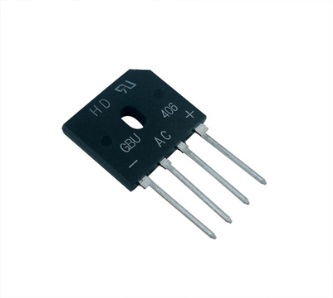Diode Bridge (Graetz)

However, in power applications, it is more common to use three-phase power sources. This occurs mainly in the industry.

For three-phase rectification we can use the diodes in the configuration shown in Figure 9.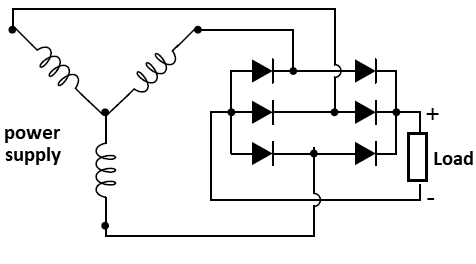Figure 9 - Three-phase Full Wave Rectifier

In this circuit, six diodes are used in a configuration in which at each instant two diodes drive.

In Figure 10, we have the pulsating current obtained in the rectifier circuit. Note that using a three-phase system, the ripple is smaller than what we find in a half-wave or full-wave two-phase rectifier system.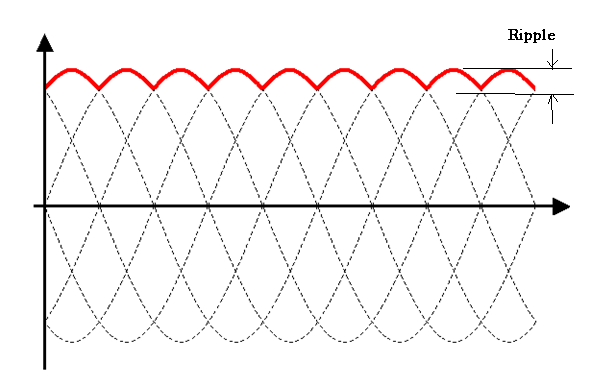Figure 10 - Three-phase rectifier input current and ripple

An interesting configuration is a six-phase rectifier system shown in Figure 11.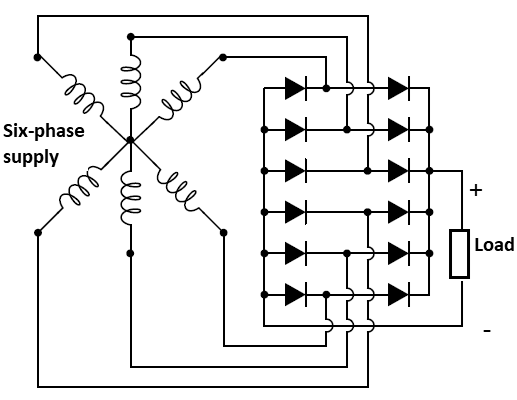Figure 11 - Six-phase Rectifier

These configurations can also be found in automobiles for rectifying the current obtained from alternators.

## Parallel Diodes

It is often thought that simply connecting diodes in parallel increases the capacity of a smooth rectifier system. However, this problem of obtaining higher current capacity is not so easily solved.

Diodes, even of the same type, do not have exactly the same characteristics. There are minor differences from one to the other and this means that if we connect diodes in parallel, the current does not divide evenly between them.

Due to slight differences in direct voltage (Vf), one diode conducts before or more than the other and the result is a difference between the currents passing through these components.

Thus, as shown in Figure 12, if we want to use two 1 A diodes to rectify a current of 2 A by connecting them in parallel, the current will be different and one of them will burn (D1) because it is overloaded.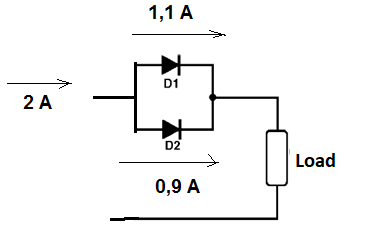Figure 12 - The current does not split evenly between diodes

To avoid this problem, connect in series with each diode a low value resistor (the lower the current intensity, the smaller), in order to better distribute the current. The diodes, as shown in Figure 13, may typically have values between 0.1 and 1 ohm for currents in the range of 1 to 5 A.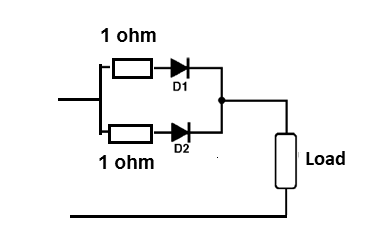Figure 13 - Distributing the current better between diodes

Note that this procedure is also adopted when we parallel transistors on voltage regulators.

## Diodes in Series

We can connect diodes in series with the purpose of achieving a higher inverse voltage. For example, theoretically two 200 V diodes can withstand an inverse voltage of 400 V.

In practice, this does not occur, because the inverse leakage current of the diodes is different, and this causes in reverse polarization the voltages to divide unevenly.

Thus, by applying 500 V to two diodes in series, it may occur that even though they are equal in specification (1N5404, for example), the inverse voltage splits so that one gets 240 V and the other 260 V and this can cause one of them to overload.

We can avoid this problem by connecting in parallel, with the appropriate value, resistor diodes which help even the split voltage. Resistors from 10k to 22k can be used in this application as shown in Figure 14.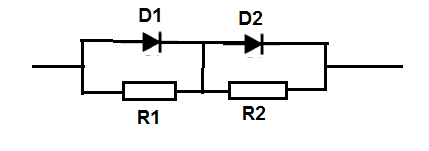Figure 14 - Using resistors to distribute voltage in diodes in series

## Current Bursts

In power supplies where after the rectifier diode we find a capacitor of very high value, a problem occurs that must be avoided, especially when the current involved is intense. When the power is turned on, the discharged capacitor behaves like a short circuit which means that a very intense current can circulate.

If this current exceeds the value of the maximum current burst (Ifsm), the diode may burn out. Although the values involved are high, because for the 3 A 1N5404, Ifsm is 200 A, at the moment the voltage is established in the circuit, if the transformer has a very large current capacity, problems may occur.

One way to avoid this problem is to connect a low value resistor in series to the diode, calculated to limit the current in the event of a short, to a value lower than Ifsm, as shown in Figure 15.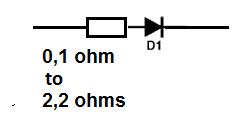Figure 15 - The diode to protect against bursts

The resistor must be of current-compatible dissipation capacity wire, remembering that the most intense current only occurs for a moment and it must be calculated so that the diode and capacitor shorted, the burst is smaller than the specified Ifsm for the component.